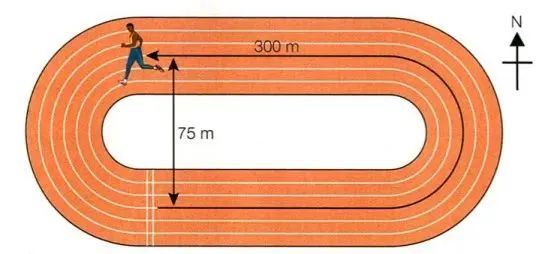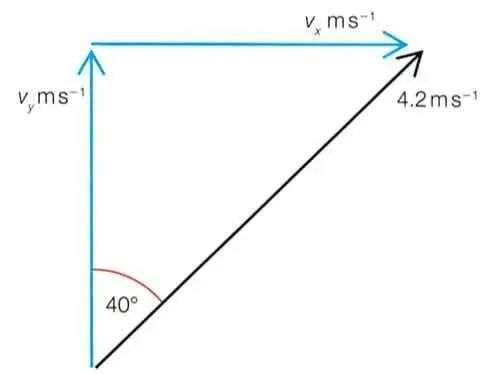# A-level物理课程试题讲解：Vector的理解和运用1、如图所示，该运动员的实际运动距离distance=300m。他的位移displacement在north方向为75m。假设该运动员所花时间为50s。

v=300/50=6m/s
velocity=displacement/time
v=75/50=1.5m/s

2、The components in figure to show how they add up to produce the 4.2m/s velocity vector, This means we can use the relationship.Cos40° = Vy/4.2
Vy=4.2 x Cos40°
=4.2 x 0.766
=3.2m/s

Sin40°=Vx/4.2
Vx=4.2 x Sin40°
=4.2 x 0.643
=2.7m/s

Alevel备考，刷题资料必不可少，扫码咨询

【免费领取】历年真题及解析，考前冲刺，名师讲解，专业答疑！